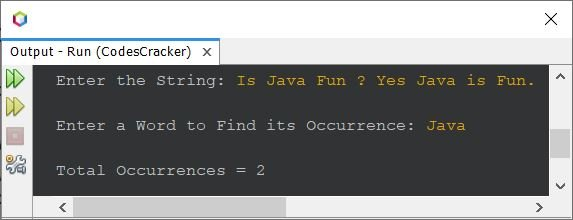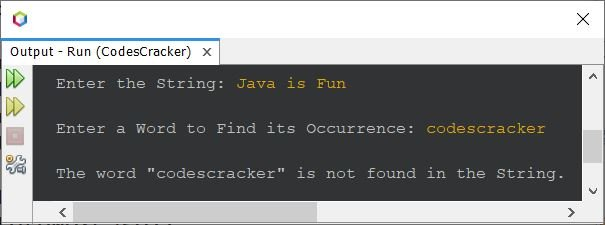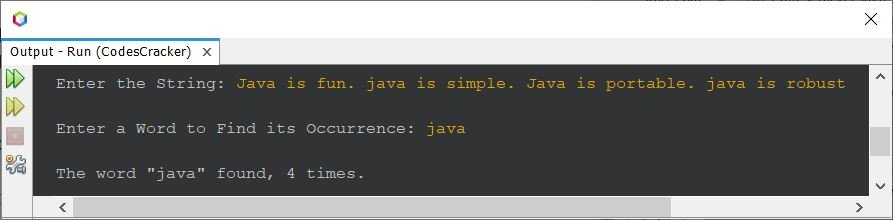# Java Program to Find Occurrence of Given Word in a String

This article is created to include a program in Java that find and prints the occurrence of a given word in given string. For example, if the given string is Java is portable, is not it? and the given word is is. Then the output will be 2, because the given word ("is") found 2 times in given string.

## Find Occurrence of a Word in String - Basic Version

The question is, write a Java program to find and print the occurrence of a word in a string. Both word and string must be received by user at run-time of the program. The program given below is its answer:

```import java.util.Scanner;

public class CodesCracker
{
public static void main(String[] args)
{
String str, word;
int wordsLen, i, count=0;
Scanner s = new Scanner(System.in);

System.out.print("Enter the String: ");
str = s.nextLine();
System.out.print("\nEnter a Word to Find its Occurrence: ");
word = s.next();

String words[] = str.split(" ");
wordsLen = words.length;

for(i=0; i<wordsLen; i++)
{
if(word.equals(words[i]))
count++;
}

System.out.println("\nTotal Occurrences = " +count);
}
}```

The snapshot given below shows the sample run of above Java program on finding the occurrence of a given word in given string, with user input Is Java Fun ? Yes Java is Fun. as string and Java as word:## Find Occurrence of a Word in String - Complete Version

Since there are multiple limitations of above program. For example:

• what if user enters a word in lowercase, and the same word is available in string, but in uppercase ?
• what if user enters a string that contains multiple white spaces in between any two or multiple words ?
• what if user enters a word that does not found in the string ?

Therefore, keeping in mind, these limitations, I've created another program that does the same job as of previous program. Also the program provides little good user experience than previous one. Let's have a look at the program given below:

```import java.util.Scanner;

public class CodesCracker
{
public static void main(String[] args)
{
String str, word;
int wordsLen, i, count=0;
Scanner s = new Scanner(System.in);

System.out.print("Enter the String: ");
str = s.nextLine();
System.out.print("\nEnter a Word to Find its Occurrence: ");
word = s.next();

str = str.toLowerCase();
word = word.toLowerCase();

String words[] = str.split("\\s+");
wordsLen = words.length;

for(i=0; i<wordsLen; i++)
{
if(word.equals(words[i]))
count++;
}

if(count==0)
else if(count==1)
System.out.println("\nThe word \"" +word+ "\" occurs only one time.");
else
System.out.println("\nThe word \"" +word+ "\" found, " +count+ " times.");
}
}```

Here is its sample run with user input Java is Fun as string and codescracker as word to search:Here is another sample run with user input Java is fun. java is simple. Java is portable. java is robust as string and java as word to find and print its occurrences:Java Online Test

« Previous Program Next Program »